# 16 Results

View
Selected filters:
• OH.TMM.0006.Core.4Rating

The Calculus II course was developed through the Ohio Department of Higher Education OER Innovation Grant. This work was completed and the course was posted in February 2019. The course is part of the Ohio Transfer Module and is also named TMM006. For more information about credit transfer between Ohio colleges and universities, please visit: www.ohiohighered.org/transfer.Team LeadJim Fowler                                         Ohio State UniversityRita Ralph                                         Columbus State Community CollegeContent ContributorsNela Lakos                                       Ohio State UniversityBart Snapp                                       Ohio State UniversityJames Talamo                                  Ohio State UniversityXiang Yan                                         Edison State Community CollegeLibrarianDaniel Dotson                                    Ohio State University                     Review TeamThomas Needham                             Ohio State UniversityCarl Stitz                                            Lakeland Community CollegeSara Rollo                                         North Central State College

Subject:
Mathematics
Calculus
Material Type:
Full Course
Provider:
Ohio Open Ed Collaborative
09/26/2018Conditional Remix & Share Permitted
CC BY-NC
Rating

After completing this section, students should be able to do the following.Determine if a series converges absolutely or conditionally.Answer conceptual questions about absolute convergence

Subject:
Calculus
Material Type:
Module
Provider:
Ohio Open Ed Collaborative
07/26/2019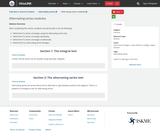Conditional Remix & Share Permitted
CC BY-NC
Rating

After completing this section, students should be able to do the following.Determine if a series converges using the alternating series test.Determine if a series converges absolutely.Determine if a series converges conditionally.Determine if an alternating series diverges.

Subject:
Calculus
Material Type:
Module
Provider:
Ohio Open Ed Collaborative
07/26/2019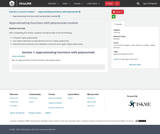Conditional Remix & Share Permitted
CC BY-NC
Rating

After completing this section, students should be able to do the following.Compute Taylor polynomials.Use Taylor’s theorem to estimate the error of a Taylor polynomial.Determine the maximum error between a function and a given Taylor polynomial.

Subject:
Calculus
Material Type:
Module
Provider:
Ohio Open Ed Collaborative
07/26/2019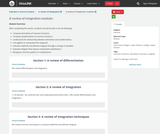Conditional Remix & Share Permitted
CC BY-NC
Rating

After completing this section, students should be able to do the following.Compute derivatives of common functions.Compute antiderivatives of common functions.Understand the relationship between derivatives and antiderivatives.Use algebra to manipulate the integrand.Evaluate indefinite and definite integrals through a change of variables.Evaluate integrals that require complicated substitutions.Recognize common patterns in substitutions.

Subject:
Calculus
Material Type:
Module
Provider:
Ohio Open Ed Collaborative
07/24/2019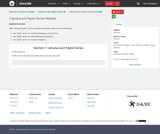Conditional Remix & Share Permitted
CC BY-NC
Rating

After completing this section, students should be able to do the following.Use Taylor series to read-off derivatives of a function.Use Taylor series to solve differential equations.Use Taylor series to compute integrals.

Subject:
Calculus
Material Type:
Module
Provider:
Ohio Open Ed Collaborative
07/26/2019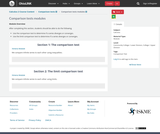Conditional Remix & Share Permitted
CC BY-NC
Rating

After completing this section, students should be able to do the following.Use the comparison test to determine if a series diverges or converges.Use the limit comparison test to determine if a series diverges or converges.

Subject:
Calculus
Material Type:
Module
Provider:
Ohio Open Ed Collaborative
07/26/2019Conditional Remix & Share Permitted
CC BY-NC
Rating

After completing this section, students should be able to do the following.Use the integral test to determine that a series diverges.Use integrals to estimate the value of a series within an error.Use the divergence test to determine that a series diverges.Manipulate series using the properties of convergent series.Recognize known convergent or divergent series.

Subject:
Calculus
Material Type:
Module
Provider:
Ohio Open Ed Collaborative
07/26/2019Conditional Remix & Share Permitted
CC BY-NC
Rating

After completing this section, students should be able to do the following.Use Taylor series to approximate constants.Use Taylor series to compute limits.Use Taylor series to sum series.

Subject:
Calculus
Material Type:
Module
Provider:
Ohio Open Ed Collaborative
07/26/2019Conditional Remix & Share Permitted
CC BY-NC
Rating

After completing this section, students should be able to do the following.Give the definition of a power series.Find the interval and radius of convergence of a power series.Express functions as power series.Express power series as closed-form functions.Differentiate and integrate power series.

Subject:
Calculus
Material Type:
Module
Provider:
Ohio Open Ed Collaborative
07/26/2019Conditional Remix & Share Permitted
CC BY-NC
Rating

The various concepts associated with sequences and series are reviewed.All of the series convergence tests we have used require that the underlying sequence (an)(an) be a positive sequence. We can actually relax this and state that there must be an N>0N>0 such that an>0an>0 for all n>Nn>N; that is, (an)(an) is positive for all but a finite number of values of nn. We’ve also stated this by saying that the tail of the sequence must have positive terms. In this section we explore series whose summation includes negative terms.

Subject:
Calculus
Material Type:
Module
Provider:
Ohio Open Ed Collaborative
07/26/2019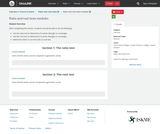Conditional Remix & Share Permitted
CC BY-NC
Rating

After completing this section, students should be able to do the following.Use the ratio test to determine if a series diverges or converges.Use the root test to determine if a series diverges or converges.Determine when to use the ratio or root test.

Subject:
Calculus
Material Type:
Module
Provider:
Ohio Open Ed Collaborative
07/26/2019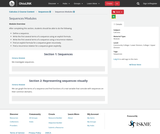Conditional Remix & Share Permitted
CC BY-NC
Rating

After completing this section, students should be able to do the following.Define a sequence.Write the first several terms of a sequence using an explicit formula.Write the first several terms of a sequence using a recurrence relation.Find an explicit formula for a sequence given recursively.Find a recurrence relation for a sequence given explicitly.

Subject:
Calculus
Material Type:
Module
Provider:
Ohio Open Ed Collaborative
07/25/2019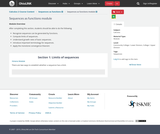Conditional Remix & Share Permitted
CC BY-NC
Rating

After completing this section, students should be able to do the following.Recognize sequences can be generated by functions.Compute limits of sequences.Understand growth rates of basic sequences.Introduce important terminology for sequences.Apply the monotone convergence theorem

Subject:
Calculus
Material Type:
Module
Provider:
Ohio Open Ed Collaborative
07/26/2019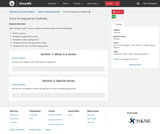Conditional Remix & Share Permitted
CC BY-NC
Rating

After completing this section, students should be able to do the following.Define a series.Recognize a geometric series.Recognize a telescoping series.Compute the sum of a geometric series.Compute the sum of a telescoping series.

Subject:
Calculus
Material Type:
Module
Provider:
Ohio Open Ed Collaborative
07/26/2019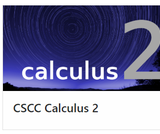Unrestricted Use
CC BY
Rating

This is a Calculus II interactive textbook with modules curated and created on The Ohio State University's Ximera platform.

Subject:
Mathematics
Calculus
Material Type:
Textbook
Provider:
Columbus State Community College
Author:
Kevin James
Lee Wayand
The Ximera Project
Tzy-Yi "Alan" Yang